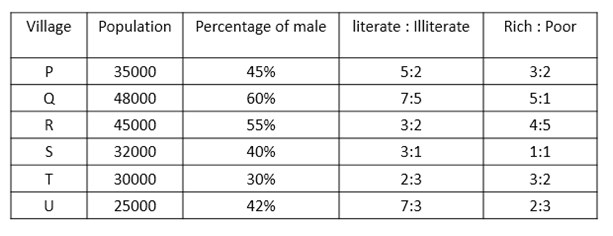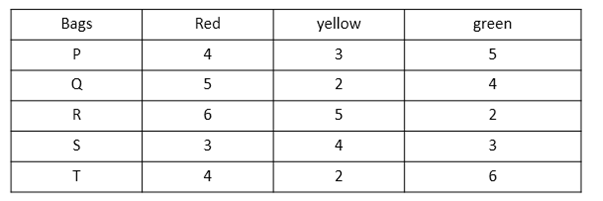# Quantitative Aptitude Questions (Data Interpretation) for SBI Clerk 2018 Day-12

## Quantitative Aptitude Questions (Data Interpretation) for SBI Clerk 2018 Day-12:

Dear Readers, SBI is conducting Online preliminary Examination for the recruitment of Clerical Cadre. preliminary Examination of SBI Clerk was scheduled from March 2018. To enrich your preparation here we have providing new series of Data Interpretation – Quantitative Aptitude Questions. Candidates those who are appearing in SBI Clerk Prelims Exam can practice these Quantitative Aptitude average questions daily and make your preparation effective.

Click “Start Quiz” to attend these Questions and view Solutions

## Daily Practice Test Schedule | Good Luck

 Topic Daily Publishing Time Daily News Papers & Editorials 8.00 AM Current Affairs Quiz 9.00 AM Logical Reasoning 10.00 AM Quantitative Aptitude “20-20” 11.00 AM Vocabulary (Based on The Hindu) 12.00 PM Static GK Quiz 1.00 PM English Language “20-20” 2.00 PM Banking Awareness Quiz 3.00 PM Reasoning Puzzles & Seating 4.00 PM Daily Current Affairs Updates 5.00 PM Data Interpretation / Application Sums (Topic Wise) 6.00 PM Reasoning Ability “20-20” 7.00 PM English Language (New Pattern Questions) 8.00 PM General / Financial Awareness Quiz 9.00 PM

Directions (Q. 1 – 5): Study the following information carefully and answer the questions given below:1. If out of females of village Q, 30% are literate, find the number of literate males of village Q.
1. 32400
2. 21340
3. 22240
4. 20040
5. none of these
1. Total number of rich population of village P is what percent of total number of females of village T?
1. 200%
2. 100%
3. 50%
4. 150%
5. none of these
1. Find out the total number of rich population from all the villages together?
1. 14000
2. 135000
3. 120000
4. 125000
5. none of these
1. If the Ratio of literate males to literate females of village S is 2:3, Find the number of illiterate males of village S.
1. 3200
2. 3500
3. 3800
4. 4200
5. none of these
1. Literate population of village R is what percent more than the poor population of village U?
1. 45%
2. 30%
3. 60%
4. 80%
5. none of these

Directions (Q. 6 -10): Study the following information carefully and answer the questions given below:1. If three balls are drawn at random from bag Q, What is the probability that all the balls are of different colours?
1. 3/11
2. 4/33
3. 8/33
4. 4/11
5. none of these
1. Total number of red balls and yellow balls together are what percent of total number of green balls?
1. 150%
2. 160%
3. 180%
4. 190%
5. None of these
1. If four balls are drawn at random from bag R, find the probability that all the balls are not of yellow colour?
1. 15/143
2. 14/143
3. 12/143
4. 17/143
5. none of these
1. Three balls are drawn from each of the bags S and T. Find the ratio of that out of three balls 2 are yellow from bag S and all the balls from bag T are green.
1. 11:10
2. 33:10
3. 43:11
4. 35:33
5. none of these
1. If three balls are drawn from both the bags P and R, find the product of their probabilities that all the balls drawn from both the bags are of different colours.
1. 90/1573
2. 90/1373
3. 99/1573
4. 90/1373
5. none of these

Directions (Q. 1 – 5):

total number of females of village Q = 40/100 x 48000 = 19200

number of literate females = 30/100 x 19200 = 5760

total number of literate population of village Q = 7/12 x 48000 = 28000

number of literate males = 28000 – 5760 = 22240

Total number of rich population of village P = 3/5 x 35000 = 21000

Total number of females of village T = (100 – 30)/100 x 30000 = 21000

Required percentage = 21000/21000 x 100 = 100%

Rich population of:

P = 3/5 x 35000 = 21000

Q = 5/6 x 48000 = 40000

R = 4/9 x 45000 = 20000

S = 1/2 x 32000 = 16000

T = 3/5 x 30000 = 18000

U = 2/5 x 25000 = 10000

Required sum = 21000 + 40000 + 20000 + 16000 + 18000 + 10000 = 125000

number of males of village S = 40/100 x 32000 = 12800

number of literate population of village S = ¾ x 32000 = 24000

number of literate males = 2/5 x 24000 = 9600

number of illiterate males = 12800 – 9600 = 3200

literate population of village R = 3/5 x 45000 = 27000

poor population of village U = 3/5 x 25000 = 15000

required percentage = (27000 – 15000)/15000 x 100 = 80%

Directions (Q. 6 -10):

Required probability = (5c1 x 2c1 x 4c1)/11c3

= (5 x 2 x 4)/165 = 8/33

total number of red balls = 4 + 5 + 6 + 3 + 4 = 22

total number of yellow balls = 3 + 2 + 5 + 4 + 2 = 16

total number of green balls = 5 + 4 + 2 + 3 + 6 = 20

required percentage = (22 + 16)/20 x 100 = 190%

Required probability = 8c4/13c4

=(8*7*6*5)/(13*12*11*10)=14/143

probability that out of 3 balls from bag S, 2 are yellow = (4c2 x 6c1)/10c3 = 36/ 120 = 3/10

probability that all the balls from bag T are green = 6c3/12c3 = 1/11

required ratio = 3/10:1/11 = 33:10

Probability of all the balls drawn from bag P are of different colours = (4c1 x 3c1 x 5c1 )/12c3

= (4 x 3 x 5)/220

= 3/11

Probability of all the balls drawn from bag R are of different colours = (6c1 x 5c1 x 2c1 )/13c3

= (6 x 5 x 2)/286

= 30/143

Required product = 3/11 x 30/143 = 90/1573